# Elephant Nose Carpet Fractal

## Project Idea

For my carpet fractal, I removed three squares for my level 1,  which are (1,2), (2,2) and (1,3). The pictures below show from level 1 to level 3. It looks like an elephant nose of “L”.

Below images are my prints of four levels.

### Level 1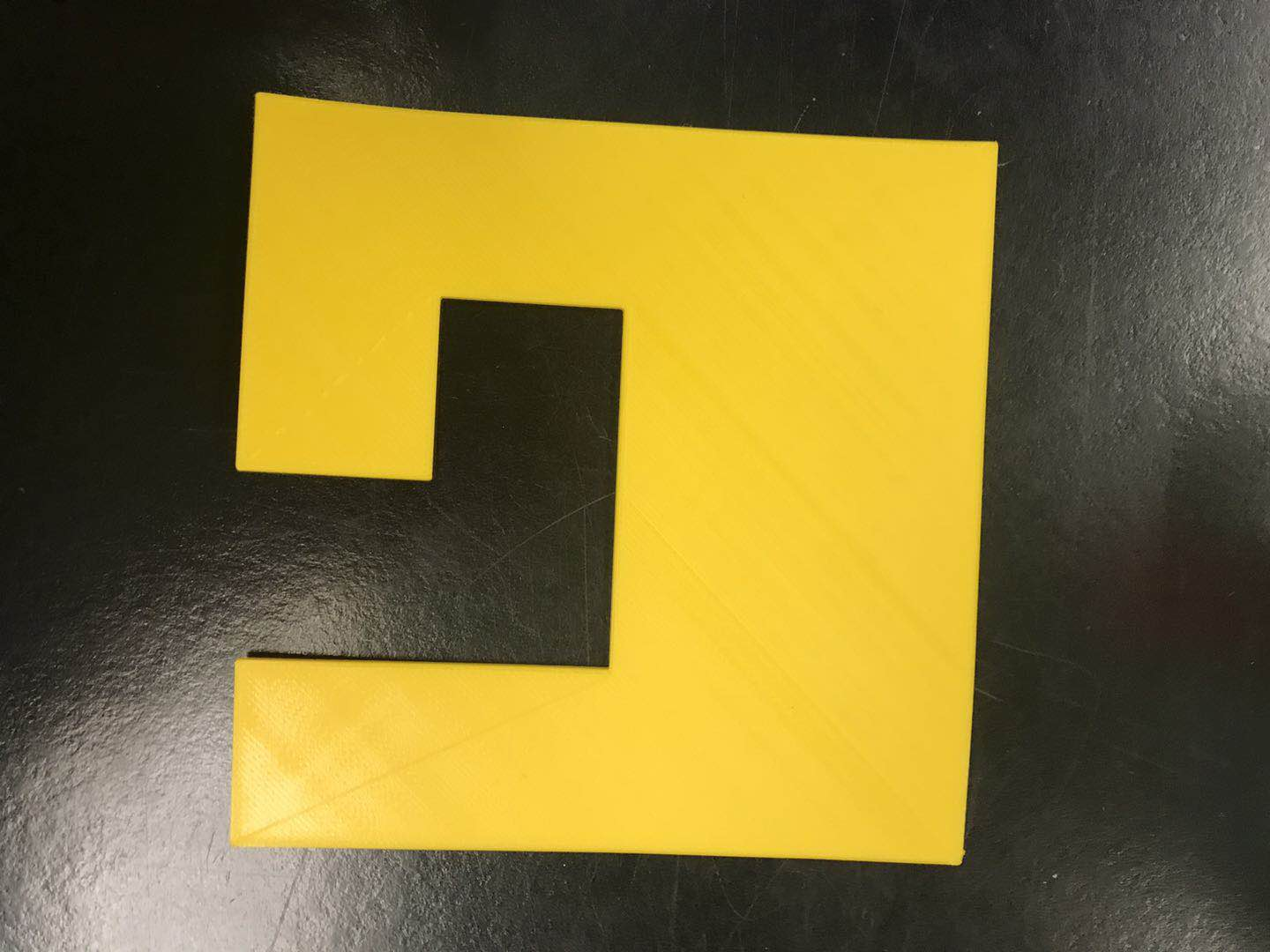### Level 2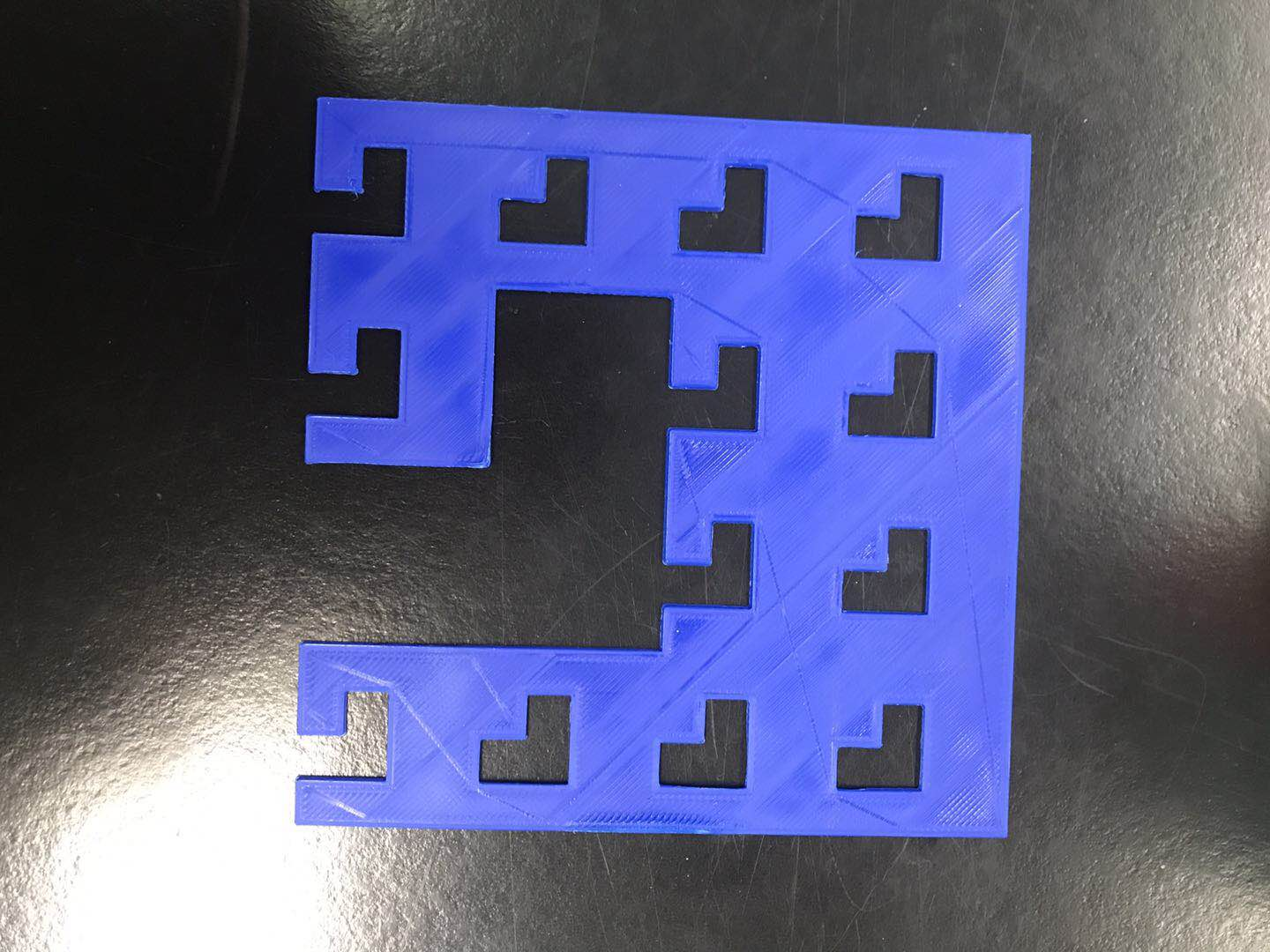### Level 3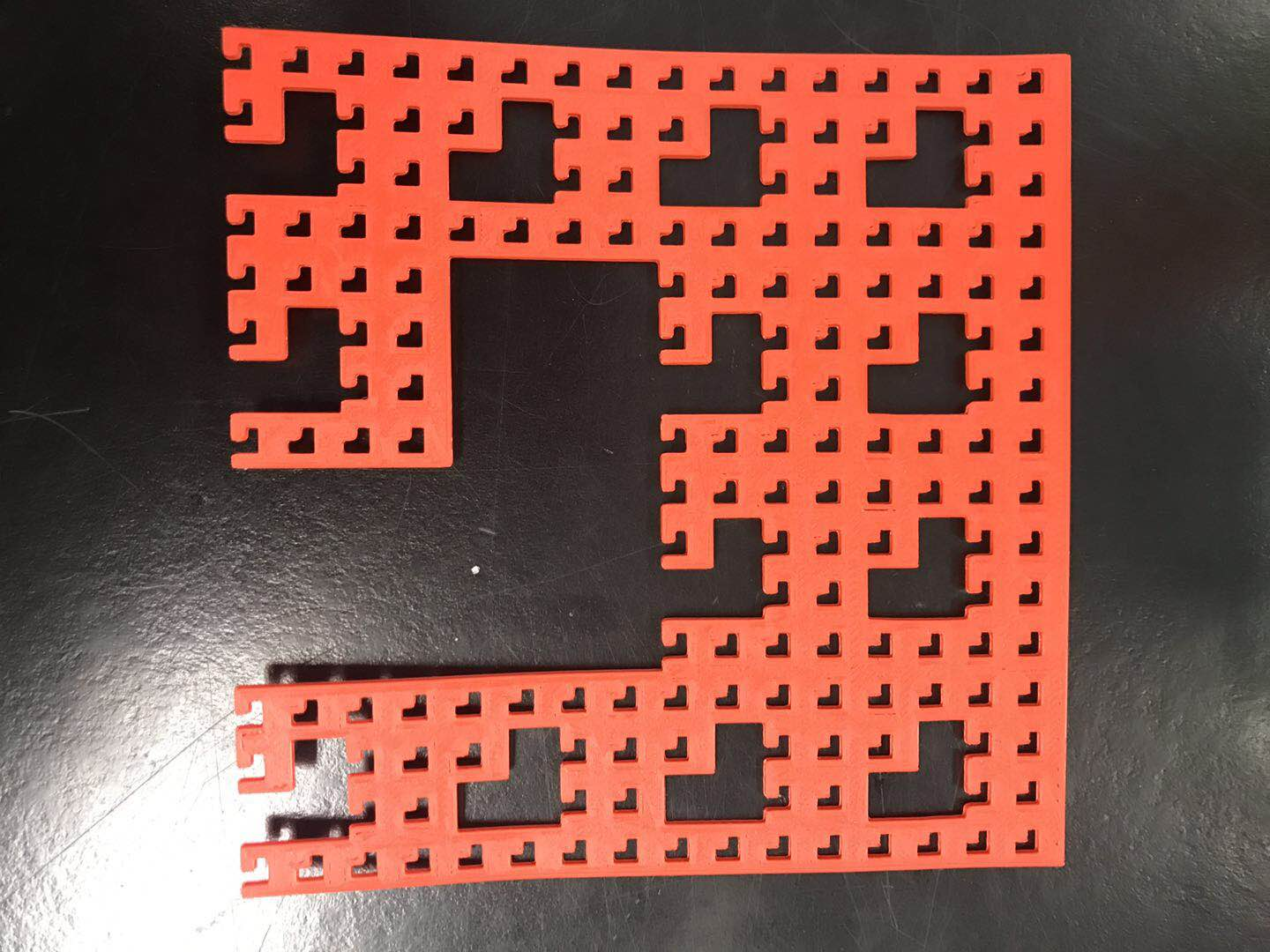### Level 4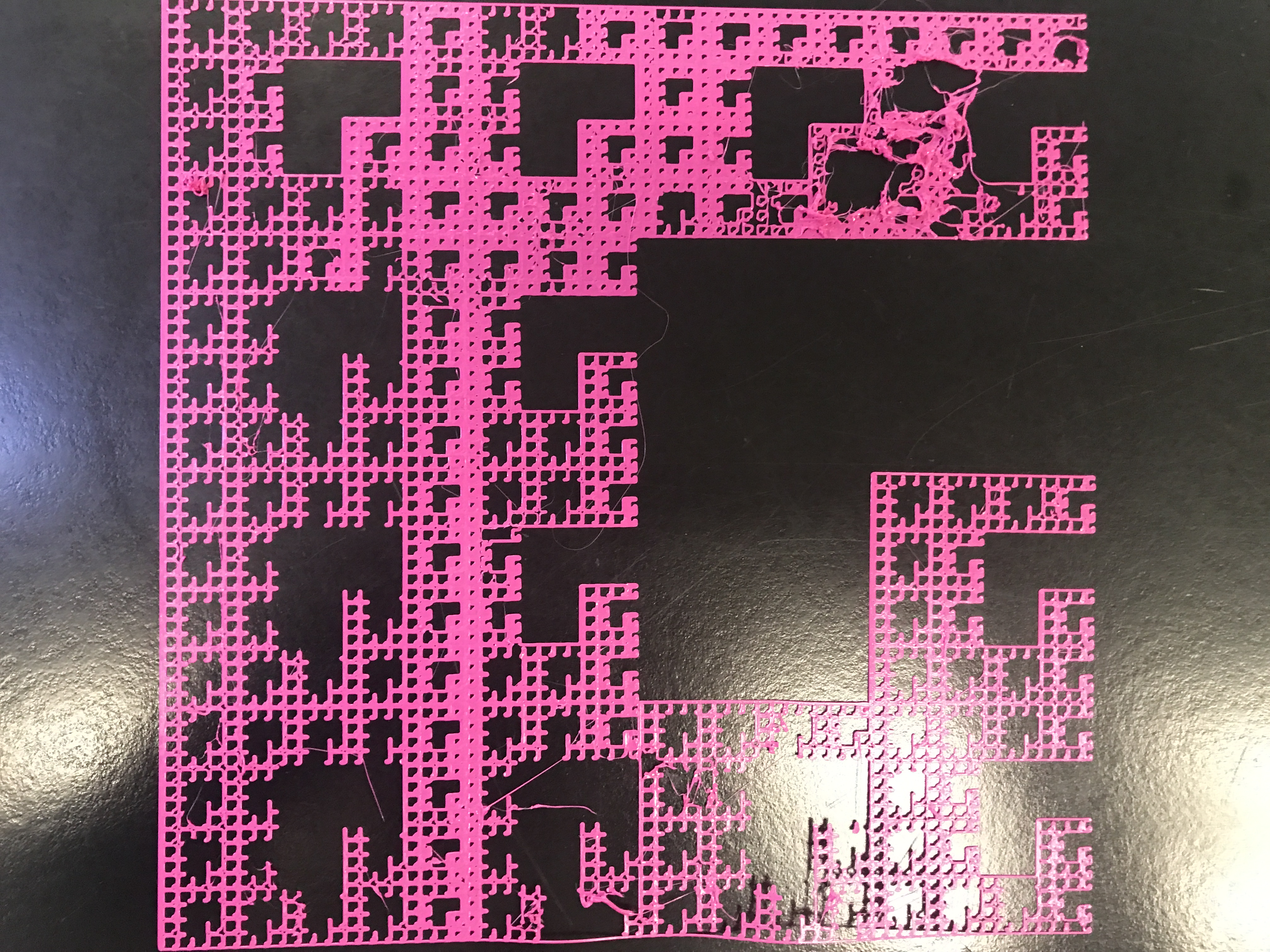## Math Calculation

Below images show the formula and area that is used for each level.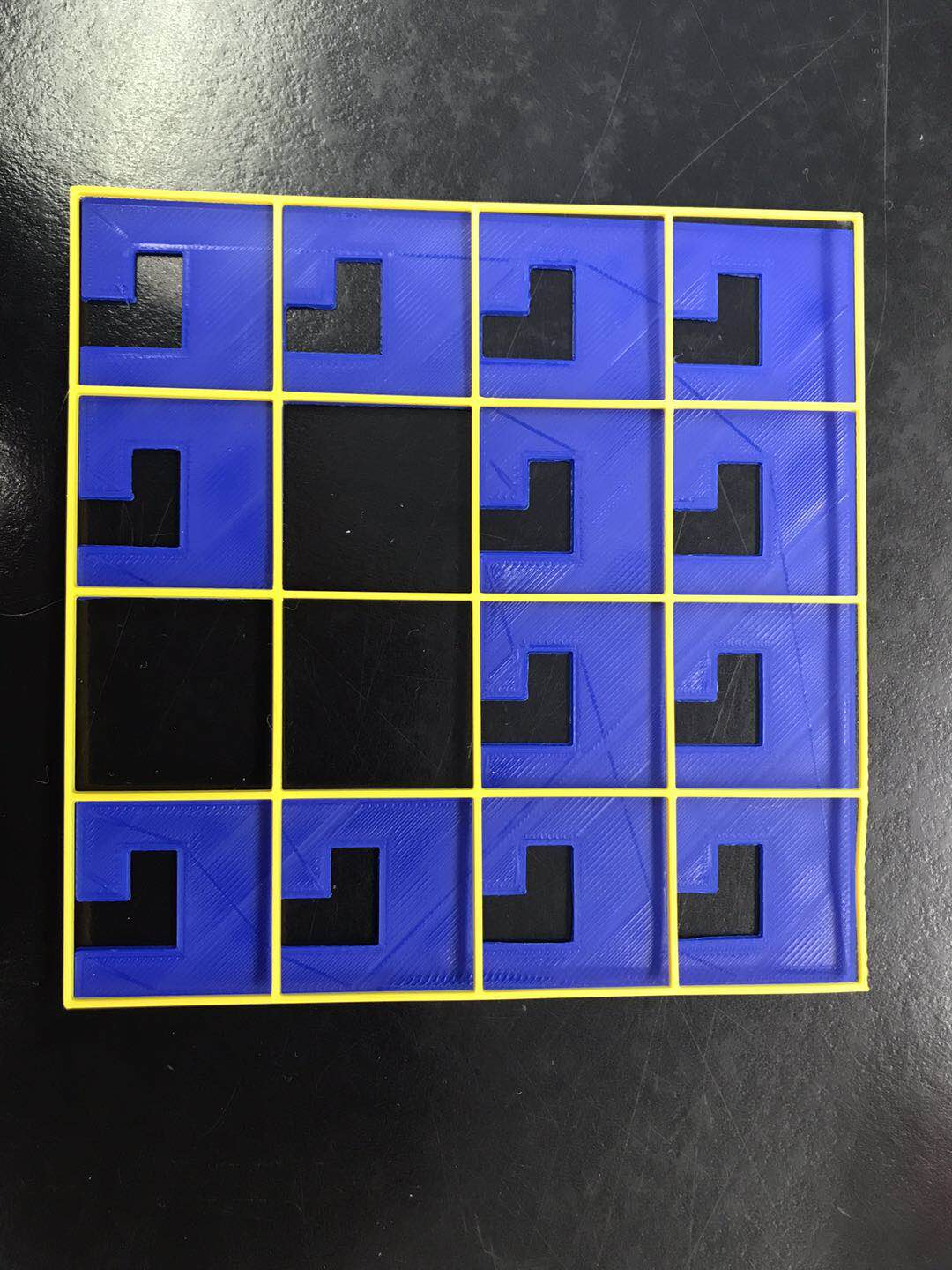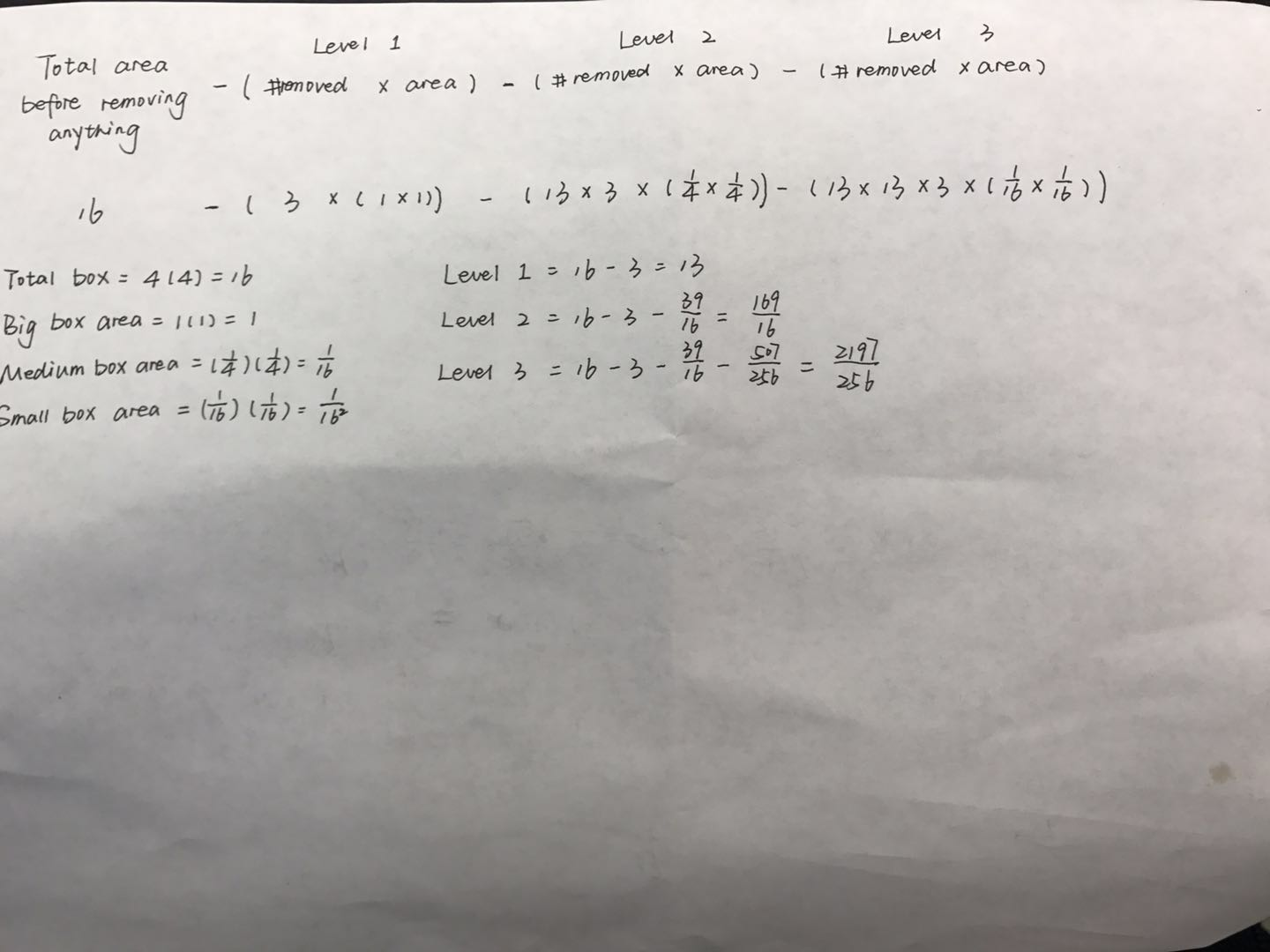Above is the area of three levels that I had. And we divide each square into 4*4.

For level 1, the length of the large square is 4, so the length of each large box is 1. The area of large box is 1*1=1

For level 2, the length of the large box is 1, so the length of each medium box is 1/4. The area of medium box 1/4 *1/4=1/16

For level 3, the length of the medium box is 1/4, so the length of each small box is 1/16. The area of small box 1/16 *1/16＝（1/16）^2

For each level, I just use total area before moving minus the area that removed. However, for next level, three squares have been moved. So we need to multiply by 13 with area each time.

Like the formula, I write down on the paper.

Level 1: area of removed= 3*(1*1)

Level 2:  area of removed=3*13*(1/4*1/4)

Level 3: area of removed=3*13*13*(1/16*1/16)

## Geometric Series

In level 1, we removed three large boxes, so it was left 13 (16-3) large boxes.

In level 2, we removed three medium boxes, so the number of medium boxes removed is 3*13=39. It times the area of medium box, which is 13*3*1/16

In level 3, we removed three small boxes, so the number of small boxes removed is 3*13*13. It times the area of small boxes, which is 13^2*3*1/16^2

All above.

We can start finding the formula for the geometric series of my carpet fractal.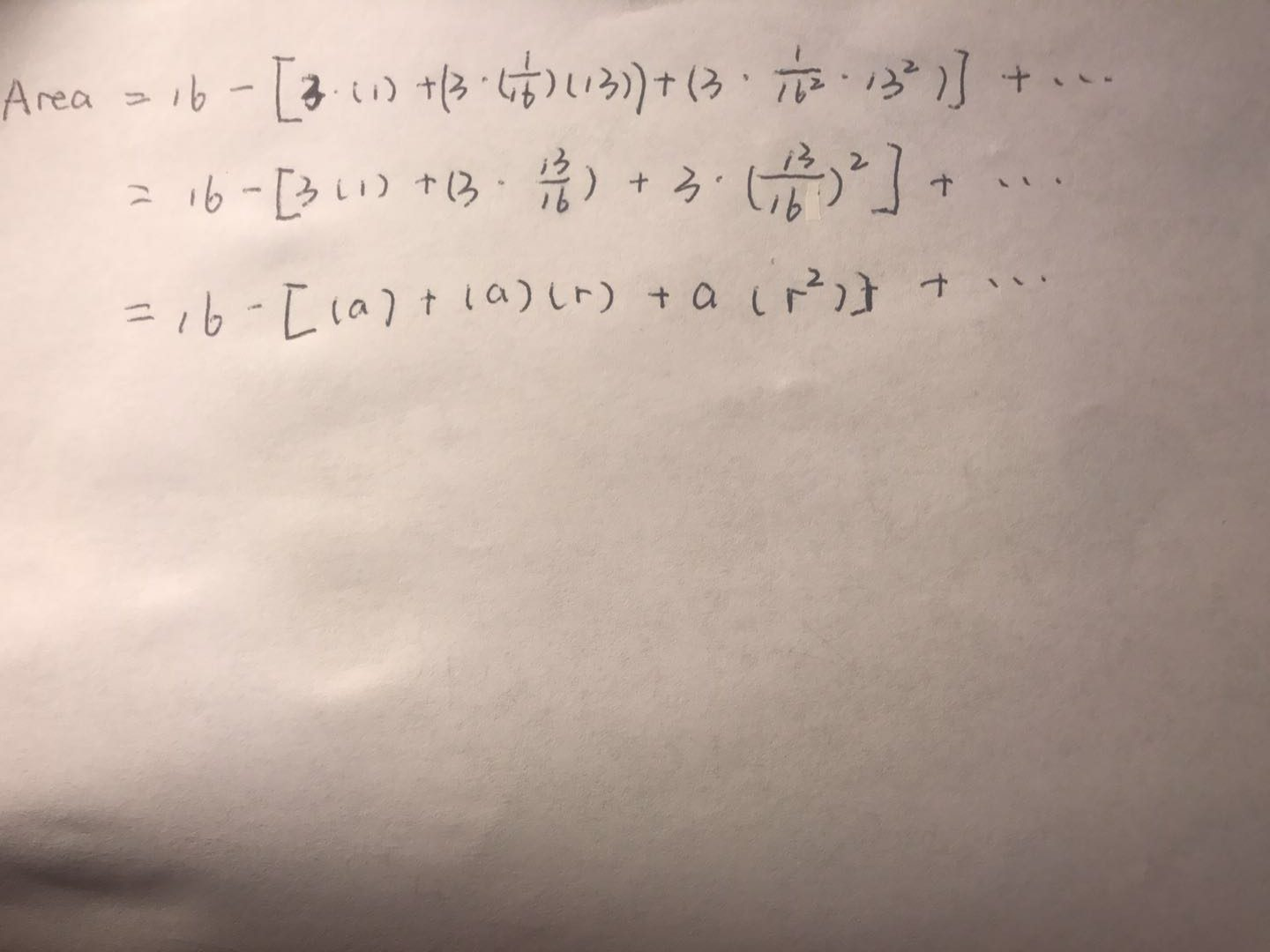## Sum Calculations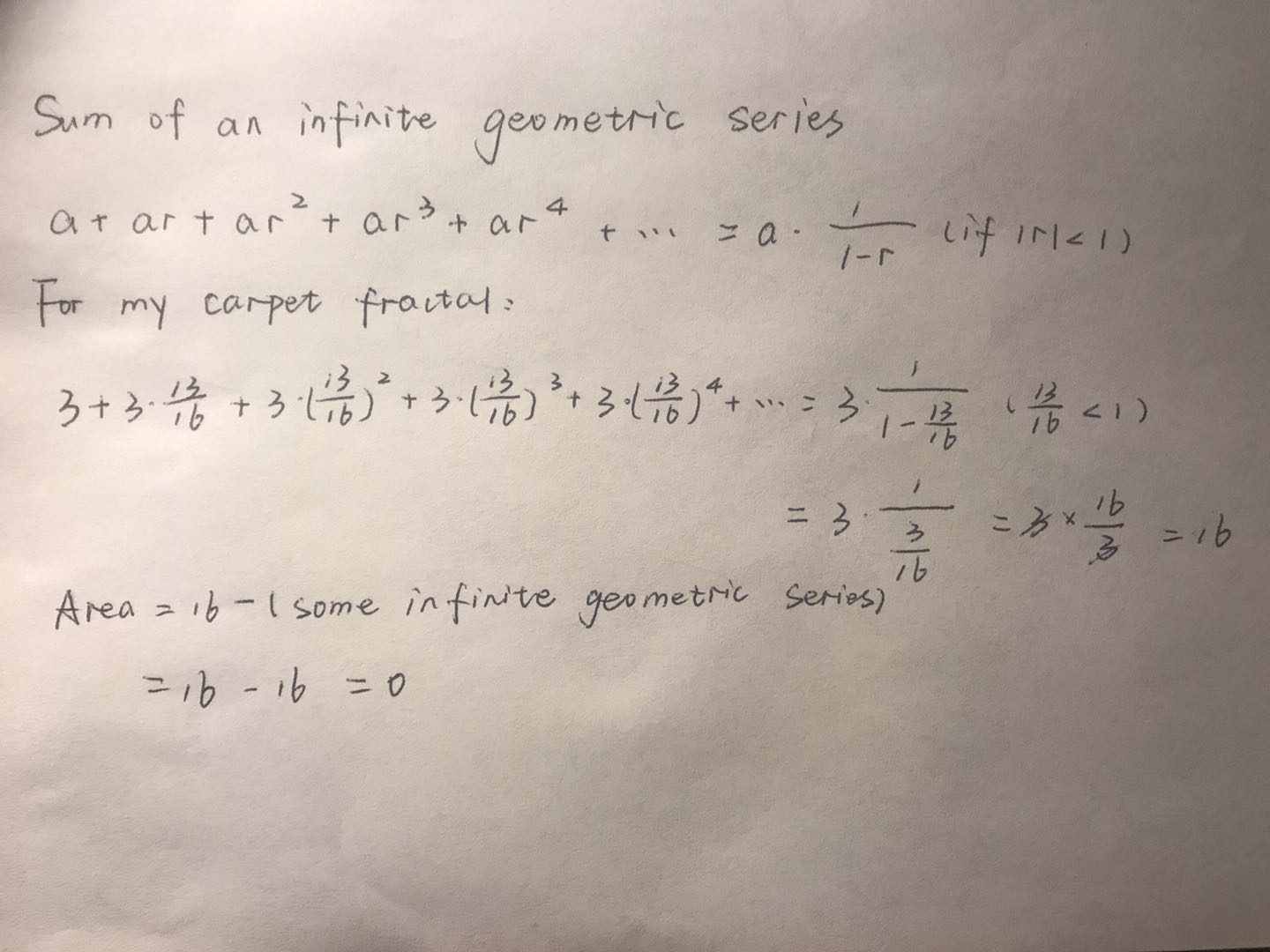We can conclude the formula sum of an infinite geometric series. To find the formula of my carpet fractal, I use the calculation above to get the finite answer of 16. At last, we use the area of the original square, which is 16 minus the sum of 16, equals to 0. So the area of infinity level is zero.

## Dimension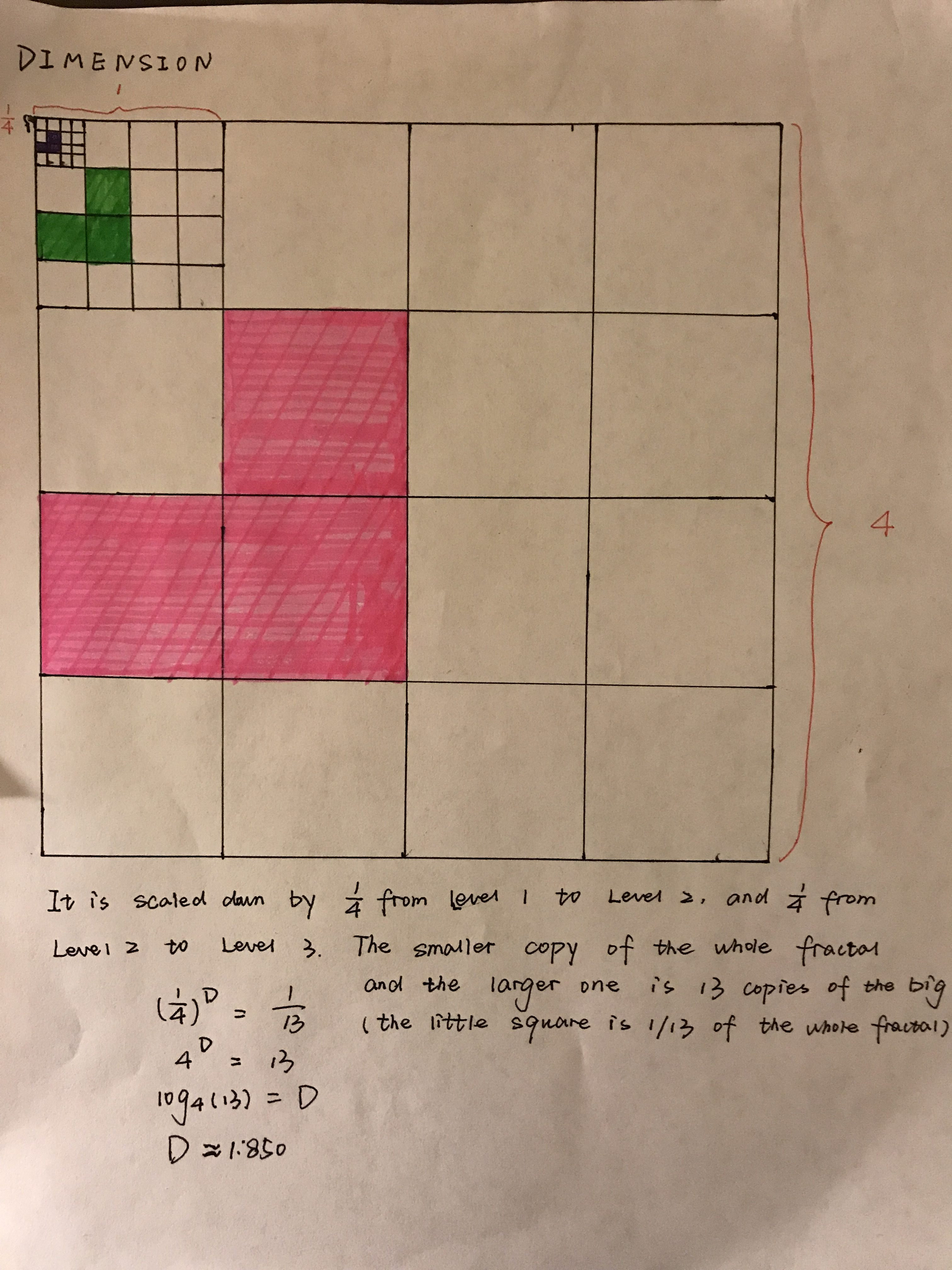It is scaled down by 1/4 from Level 1 to Level 2, and the same as Level 2 to Level 3. As I marked, the smaller copy of the whole fractal and the larger one is 13 copies of the big one. For my fractal, like the different colors I marked in different levels, I cut down three squares in each box. Then each level left 13 squares among the bigger square. After that, It means the little square is 1/13 of the whole fractal.

Above, I use the formula to calculate dimension, which is (1/4) ^D=1/13.

As I write down on the paper, I use log formula to get Dimension. At last, it came out the dimension is D=1.850.